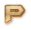Python Numeric Types Online Test

This Python Numeric Types Online Test helps you to Boost your Knowledge in Python. In this test, we will cover the topics in the form of questions like

• We cannot compare the floating data type using equal to operator.
• l is used to determine long. We cannot use it in complex number.
• inf or Infinity is the unique case of floating data type and it is defined as float(inf).
• When we use 0 before any number that is the representation of octal number but octal number only contain numbers from 0 to 7, not 9.
• ^ is used as XOR operator in Python.

Instructions:
• Total number of questions : 9.
• Time alloted : 10 minutes.
• Each question carry 1 mark.
• No Negative marks
• DO NOT refresh the page.
• All the best :-)

What You Will Learn in

This Python Numeric Types Online Test helps you to Boost your Knowledge in Python. In this test, we will cover the topics in the form of questions like

• We cannot compare the floating data type using equal to operator.
• l is used to determine long. We cannot use it in complex number.
• inf or Infinity is the unique case of floating data type and it is defined as float(inf).
• When we use 0 before any number that is the representation of octal number but octal number only contain numbers from 0 to 7, not 9.
• ^ is used as XOR operator in Python.